# Computing GIS Summaries from Map Unit Polygons

D.E. Beaudette
2016-08-17

A report that performs map unit comparisons is available here.

## Introduction

This tutorial describes one approach to summarizing raster data (e.g. slope, aspect, PRISM data, etc.) according to delineations of a specific map unit. The examples outlined below are a good starting point for a more rigorous examination of map unit variability for the purposes of SDJR, quality control, MLRA update work, or DSM project. You will need to adjust file paths to raster and vector data sources below, in order to follow along with your own data.

Copy and paste blocks of code in this tutorial into a new R Studio script file (ctrl + shift + n makes a new file), edit, and then run. Running lines or blocks of code in an RStudio script file is as simple as moving the cursor to the line (or selecting a block) of code and press ctrl + enter.

## Setup R Environment

This step is only required the first time you open R. These packages will be available via `library()` in later sessions.

With a recent version of R (>= 2.15), it is possible to get all of the packages that this tutorial depends on via:

``````# run these commands in the R console, only once
install.packages('plyr', dep=TRUE)
install.packages('reshape2', dep=TRUE)
install.packages('rgdal', dep=TRUE)
install.packages('raster', dep=TRUE)
install.packages('sharpshootR', dep=TRUE) # stable version from CRAN + dependencies``````

## Map Unit Data

Map unit polygons can be loaded from shapefile, "file" geodatabase, SDA, or any other OGR-compatible data source. When working with a large number of polygons it can be advantageous to work with a subset generated using an external GIS application. In this example, the subsetting operations is performed in R. Be sure to adjust the path below to point to a file containing map unit polygons.

Specifying paths in R must be done with either a single forward slash (/) or two backslashes (\\) between directories. Specifying the path to a shapefile or other data source also requires specialized syntax:

• file geodatabase (E:/gis_data/FG_CA630.gdb)
• dsn="path_to_file/file.gdb"
• layer="feature_class_name"
• example: `readOGR(dsn='E:/gis_data/FG_CA630.gdb', layer='ca630_a', encoding='OpenFileGDB', stringsAsFactors = FALSE)`
• shapefile (E:/gis_data/ca630_a.shp)
• dsn='path_to_parent_directory' (note trailing "/" is omitted)
• layer='filename' (note trailing ".shp" is omitted)
• example: `readOGR(dsn='E:/gis_data', layer='ca630_a', stringsAsFactors = FALSE)`
• SDA (polygons loaded from the live SSURGO data via Soil Data Access)

After loading and optionally filtering-out a single map unit, a unique ID is assigned to each polygon. This ID can later be used to reference polygons that are associated with raster values outside of some ideal range.

``````# load required packages
library(rgdal)
library(raster)
library(plyr)
library(reshape2)
library(sharpshootR)
library(lattice)

# Shapefile Example
# mu <-  readOGR(dsn='E:/gis_data/ca630', layer='ca630_a', stringsAsFactors = FALSE)

# File Geodatabase Example
mu <-  readOGR(dsn='L:/CA630/FG_CA630_OFFICIAL.gdb', layer='ca630_a', encoding='OpenFileGDB', stringsAsFactors = FALSE)

# extract polygons for a single map unit ("MUSYM" attribute = "7089")
# note that column names in your data may be different
mu <- mu[which(mu\$MUSYM == '7089'), ]

# add a unique polygon ID
mu\$pID <- seq(from=1, to=length(mu))``````

## Raster Data

Raster data sources should be in a file format the can be read by the GDAL library; GeoTiFF, ArcGrid, or ERDAS formats are typically a safe option. There is currently no way to read raster data from an ESRI FileGeodatabase.

Several raster data sources are used in this example:

• "maat": 800 meter resolution, mean annual air temperature (1981--2010 PRISM) [UTM zone 10]
• "ppt": 800 meter resolution, mean annual precipitation (1981--2010 PRISM) [UTM zone 10]
• "wb": 800 meter resolution, mean of annual monthly differences between precipitation and potential ET (PRISM) [GCS NAD83]
• "elev": 10 meter resolution, USGS DEM [UTM zone 10]
• "slope": 10 meter resolution, 3x3 classic slope map derived from "elev" [UTM zone 10]
• "aspect": 10 meter resolution, 3x3 classic aspect map derived from 10 meter "elev" [UTM zone 10]

When using your own data, adjust the names (e.g. "maat") and paths (e.g. "E:/gis_data/prism/tavg_1981_2010.tif") accordingly. The use of a single forward slash (/) to separate directories is suggested.

``````raster.list <- list(maat=raster('E:/gis_data/prism/final_MAAT_800m.tif'),
ppt=raster('E:/gis_data/prism/final_MAP_mm_800m.tif'),
wb=raster('E:/gis_data/prism/effective_precipitation_800m.tif'),
)``````

Depending on the number of raster files, resolution, extent, and amount of memory on your workstation, it may be possible to load all of the rasters into RAM. It is worth trying because the performance gain can be significant. If you do not have enough memory, consider closing other applications and trying again. The following code will attempt to load all raster layers into memory, one at a time.

``````# estimate required RAM, in MB, descending order
raster.storage.mb <- sort((sapply(raster.list, ncell) * 1.1) / 1000 / 1000)
# iterate over rasters and read into memory if possible
for(i in 1:length(raster.storage.mb)) {
# update available memory
mem.available.mb <- memory.size()
# current raster
r.i <- raster.storage.mb[i]
r.i.name <- names(r.i)
# load current raster into RAM if it will fit
if(r.i < mem.available.mb) {
if(! inMemory(raster.list[[r.i.name]]) ){
print(paste0(r.i.name, ' raster loaded into RAM'))
}
}
else
print(paste0(r.i.name, ' raster NOT loaded into RAM'))
}``````
``````##  "maat raster loaded into RAM"
##  "ppt raster loaded into RAM"
##  "wb raster loaded into RAM"
##  "elev raster loaded into RAM"
##  "slope raster loaded into RAM"
##  "aspect raster loaded into RAM"``````

## Generate Sampling Points

As long as within-polygon variability (elevation, slope, aspect, etc.) is relatively low, it is possible to generate reasonable summaries of these values using a subset of those pixels that fall within each polygon. Sampling each polygon at a constant density (e.g. 1 sample per ac.) ensures that summaries of raster data are not biased by polygon size. It is important to select a sampling density based extent and size of map unit delineations relative to raster data resolution. For example, a lower density (1 sample per 10 ac.) would be sufficient for summarizing broad-scale variation in climate, while a higher density (2 samples per ac.) would be required to capture finer-scale variation in slope.

Sampling of map unit polygons is performed with the `constantDensitySampling()` function from the sharpshootR package. Relevant arguments to this function include:

• `n.pts.per.ac`: sampling density expressed as points / ac.
• `min.samples`: minimum number of samples required per polygon
• `polygon.id`: column containing a unique polygon ID

The following code will only work if the coordinate reference system of the map unit polygons and sampled raster (slope in this case) are the same. In this example the two data sets are both using the UTM zone 10, NAD83 coordinate system.

``````# sample each polygon at a constant density, 1 point per acre
s <- constantDensitySampling(mu, n.pts.per.ac=1, min.samples=1, polygon.id='pID')

# extract a single polygon and associated samples
# in this case, the second polygon
p.example <- mu[which(mu\$pID == 2), ]
s.example <- s[which(s\$pID == 2), ]

# graphical check of a single polygon
# note: polygons and raster must use the same coordinate reference system
par(mar=c(1,1,4,1))
# plotting the raster named "slope", you may need to adjust depending on your raster data
plot(raster.list[['slope']], ext=extent(p.example), axes=FALSE)
points(s.example, cex=0.75, pch=3, col='black')
box()
title('Sampling Points, Polygon #2\nSlope Raster')``````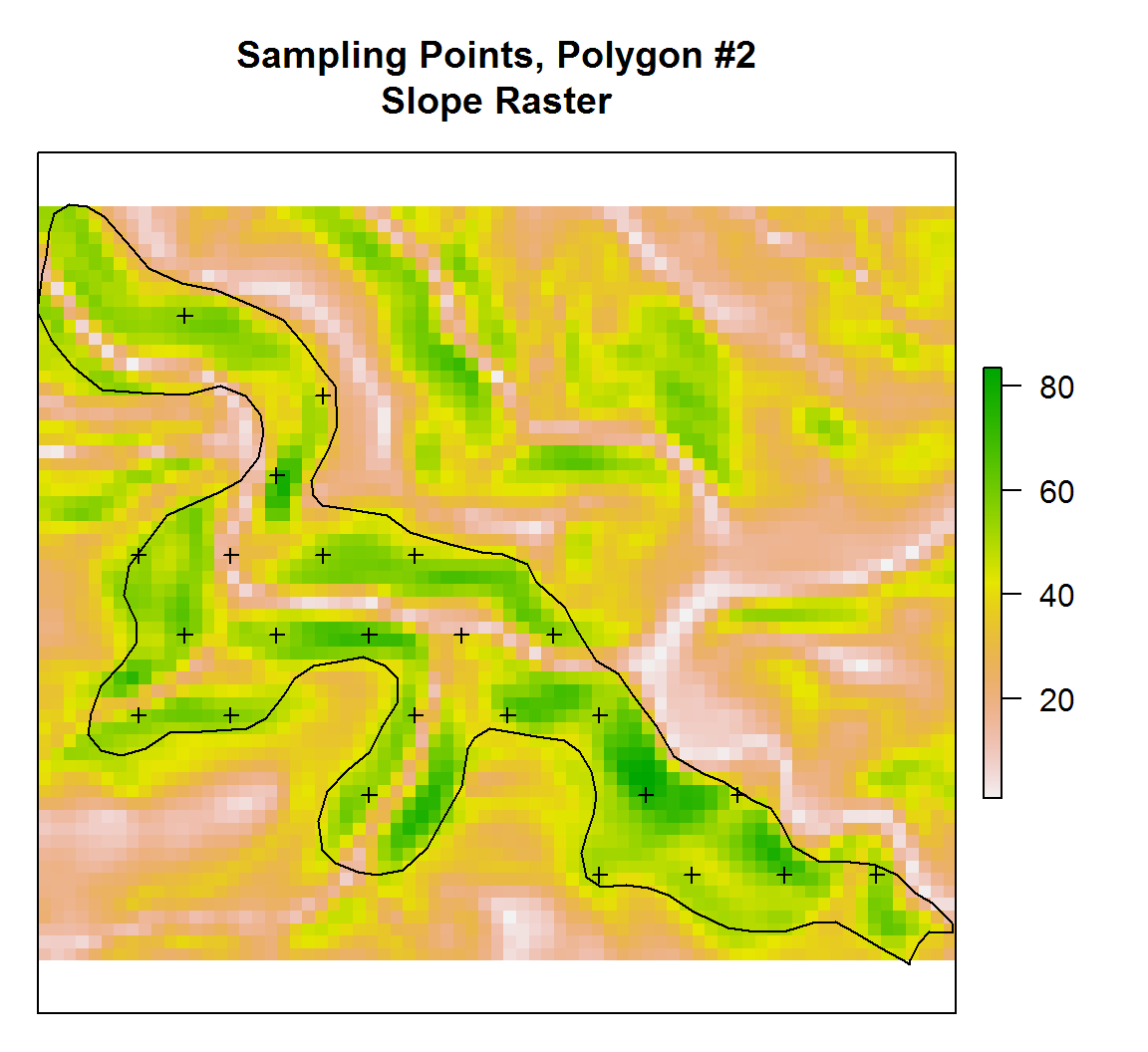## Extract Raster Data at Sample Points

The following code iterates over each raster, extracts values at sample points, and combines into a single object, `d`. Sample points are automatically transformed to the spatial reference system of each raster by the `extract()` function. Note that samples from "continuous" data sources (elev, slope, etc.) are split from "circular" data sources (aspect).

``````# initialize a list to store results
l <- list()

for(i in seq_along(raster.list)) {
i.name <- names(raster.list)[i]
l[[i.name]] <- data.frame(value=extract(raster.list[[i]], s), pID=s\$pID)
}

# convert to dataframe and fix default naming of raster column
d <- ldply(l)
names(d) <- 'variable'

# split into ratio-scale and circular stats
d.circ <- subset(d, subset=variable == 'aspect')
d <- subset(d, subset=variable != 'aspect')``````

### Check Distributions

Sometimes it is useful to see the distribution of each sampled raster. Density plots are a modern alternative to the histogram and useful for investigating univariate distributions. The x-axis units are defined by the raster layer named in the panel "strips". The y-axis units are based on relative proportion or frequency. The 5th, 50th, and 95th percentiles are printed in red, near the bottom edge of each panel.

``````densityplot(~ value | variable, data=d, scales=list(relation='free'), plot.points=FALSE, col='black', panel=function(x, ...) {
panel.densityplot(x, ...)
p.stats <- quantile(x, probs=c(0.05, 0.5, 0.95), na.rm = TRUE)
panel.text(x=p.stats, y=0, round(p.stats), pch=15, col='red')
})``````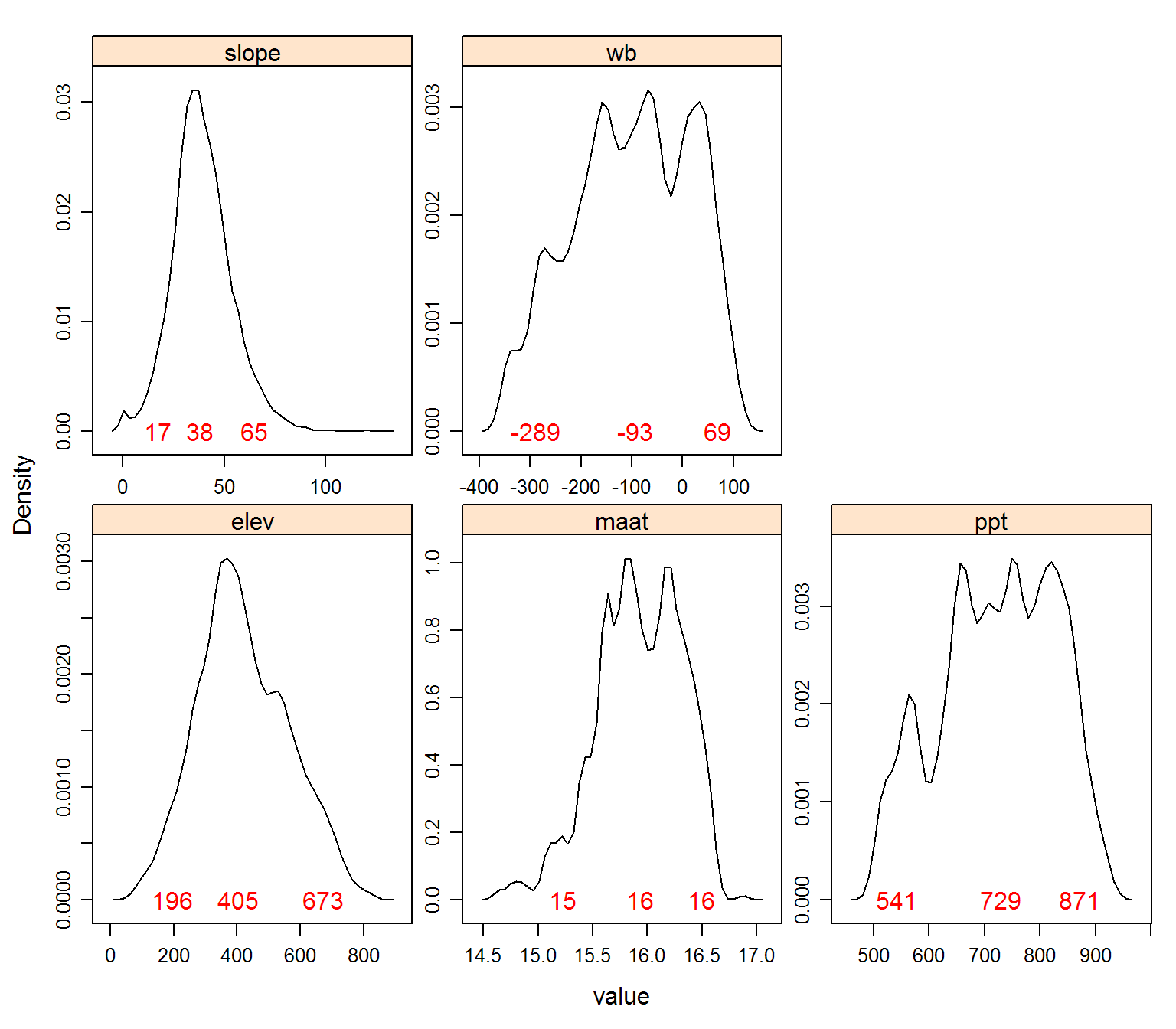## Summarize Raster Stats Over All Delineations

Quantiles are a convenient and (mostly) assumption-free method for describing distributions. In this tutorial the 5th, 25th, 50th, 75thm and 95th percentiles are used to summarize distributions. The 5th, 50th (median), and 95th percentiles are good candidates for "low", "RV", and "high" values. An estimate of variation relative to central tendency is computed using the median absolute deviation (MAD) divided by the median. This value is commonly referred to as the MADM. The natural logarithm of the absolute value of MADM yields an index of variation, scaled by central tendency, that is useful for comparison of populations that have drastically different scales. For example, precipitation in mm vs. slope in percent. Smaller values suggest less variability.

``````# define summarizing function
summary.function <- function(i, p=c(0.05, 0.25, 0.5, 0.75, 0.95)) {
# remove NA
v <- na.omit(i\$value)
# compute quantiles
q <- quantile(v, probs=p)
q <- round(q)
res <- data.frame(t(q))
# compute spread around central tendency, but only if there are > 1 samples
if(nrow(res) > 0) {
# take the natural log of absolute values of MADM
# 0's become -Inf: convert to 0

# assign reasonable names (quantiles)
names(res) <- c(paste0('Q', p * 100), 'log_abs_madm')
return(res)
}
# return NULL if there are < 1 samples
else
return(NULL)
}

# apply summary function to samples collected from each raster
stats <- ddply(d, 'variable', summary.function)

# print stats to screen
stats``````
variable Q5 Q25 Q50 Q75 Q95 log_abs_madm
elev 196 322 405 522 673 -1.0164445
maat 15 16 16 16 16 -3.6253382
ppt 541 652 729 810 871 -1.8284286
slope 17 30 38 48 65 -1.0719821
wb -289 -184 -93 3 69 0.3890639

## Summarize Raster Stats by Polygon

Sometimes it is useful to summarize raster data for each polygon. The summary function defined above is used here as well.

``````# compute summaries
poly.stats <- ddply(d, c('pID', 'variable'), summary.function)

# convert to wide format, keeping median value
poly.stats.wide.1 <- dcast(poly.stats, pID ~ variable, value.var = 'Q50')
# convert to wide format, keeping log_abs_madm
poly.stats.wide.2 <- dcast(poly.stats, pID ~ variable, value.var = 'log_abs_madm')

# add a suffix to variable names so that we can combine
names(poly.stats.wide.1)[-1] <- paste0(names(poly.stats.wide.1)[-1], '_Q50')

# join median + QCD stats for each polygon
poly.stats.wide <- join(poly.stats.wide.1, poly.stats.wide.2, by='pID')

# check first 10 rows
1 475 16 788 34 -21 -2.5060876 0.000000 0.000000 -1.0539954 0.0000000
2 230 16 528 52 -330 -2.5052909 0.000000 0.000000 -2.3416933 0.0000000
3 277 16 528 32 -323 -2.6786328 -6.205864 -5.875299 -1.7827192 -4.9215707
4 367 16 562 35 -270 -2.2008420 0.000000 0.000000 -1.6449687 0.0000000
5 226 16 553 34 -250 -0.7305545 -4.273660 -3.282503 -0.8487543 -1.5952534
6 235 16 570 37 -280 -1.6764530 -4.935053 -4.853227 -0.9486596 -3.1333169
7 524 15 846 37 62 -3.4823252 -5.373222 -4.554963 -1.5229250 -3.3211210
8 513 15 687 49 -130 -1.5949321 -3.470742 -3.003043 -1.2643015 -0.8670718
9 418 16 760 41 -78 -1.5298196 0.000000 0.000000 -1.0494512 0.0000000
10 550 16 799 33 -15 -2.8502639 -3.996236 -4.343654 -1.9005605 0.8191804

Save to CSV file for later use.

``write.csv(poly.stats.wide, file='poly-stats.csv', row.names=FALSE)``

Join polygon ID and raster statistics with original map unit polygon layer and save to SHP file.

``````# join stats to map unit polygon attribute table
mu@data <- join(mu@data, poly.stats.wide, by='pID', type='left')
# save to file
writeOGR(mu, dsn='.', layer='polygons-with-stats', driver='ESRI Shapefile', overwrite_layer=TRUE)``````

## Summarize Aspect Angle Over All Delineations

Aspect angle is a circular value and thus requires a specialized approach to computing quantiles. A graphical depiction helps with interpretation.

``````par(mar=c(0,0,0,0))
circ.stats <- aspect.plot(d.circ\$value, q=c(0.05, 0.5, 0.95), plot.title='7089', pch=NA, bg='RoyalBlue', col='black', arrow.col=c('grey', 'red', 'grey'), stack=FALSE, p.bw=90)``````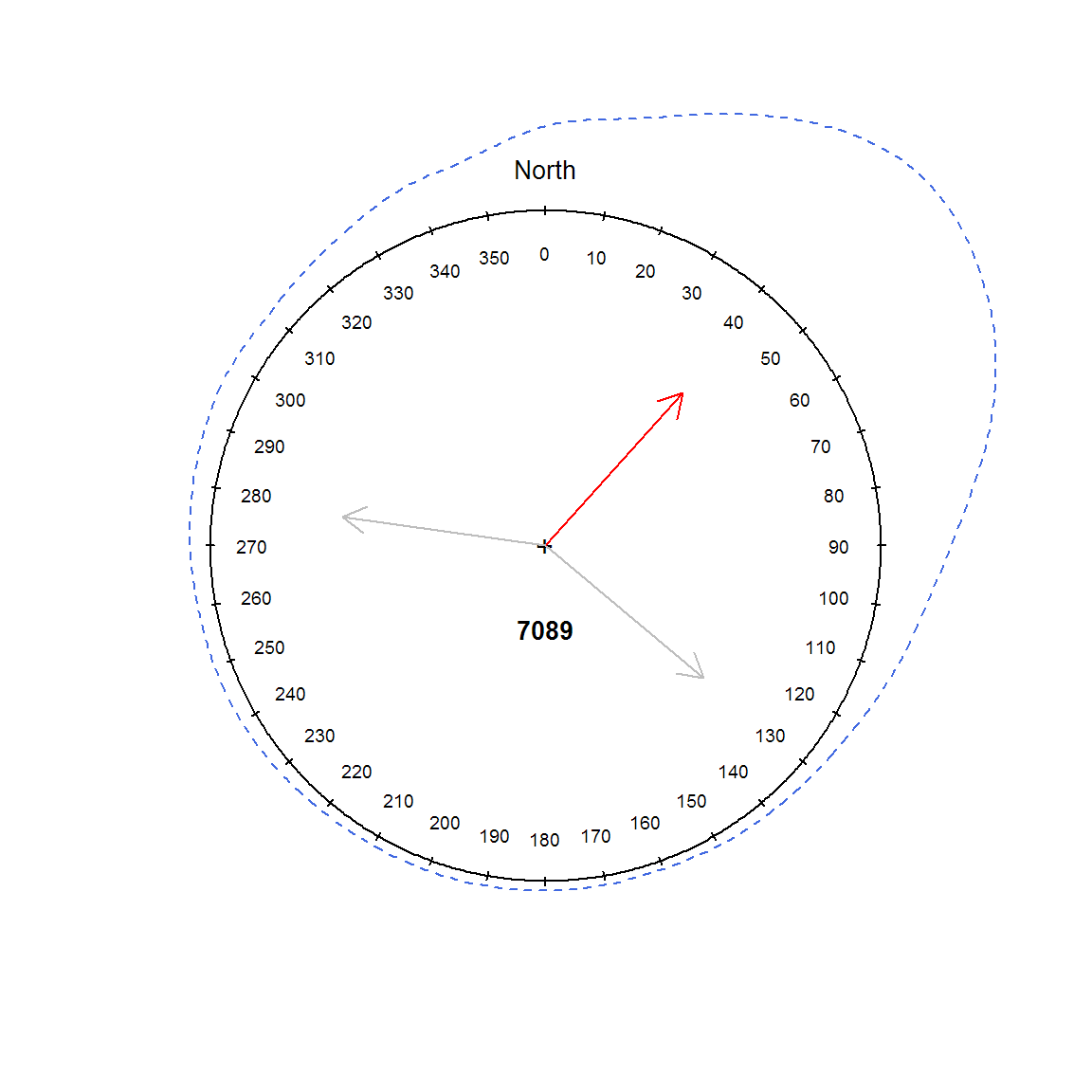``print(round(circ.stats))``
``````## Circular Data:
## Type = angles
## Units = degrees
## Template = geographics
## Modulo = 2pi
## Zero = 1.570796
## Rotation = clock
##  5% 50% 95%
## 130  42 278``````

The following code chunks highlight some additional ways in which the sampled raster values can be used to perform quality control or gain additional insight into map unit variability.

Locate polygons with >15% of samples outside of 5th-95th percentile range.

``````# define function to check for polygons with p.crit fraction of samples outside of range
f.check <- function(i, p.crit=0.15) {

# convert to values -> quantiles
e.i <- ecdf(i\$value)
q.i <- e.i(i\$value)
# locate those samples outside of our 5th-95th range
out.idx <- which(q.i < 0.05 | q.i > 0.95)

## TODO: may need to protect against no matching rows?
tab <- sort(prop.table(table(i\$pID[out.idx])), decreasing = TRUE)
df <- data.frame(pID=names(tab), prop.outside.range=round(unlist(tab), 2))
# keep only those with > 15% of samples outside of range
df <- df[which(df\$prop > p.crit), ]

return(df)
}

# apply across raster values, to all polygons
polygons.to.check <- ddply(d, 'variable', f.check)

# print details on flagged polygons
polygons.to.check``````
variable pID prop.outside.range
elev 5 0.29
elev 47 0.23
maat 5 0.27
maat 8 0.24
ppt 93 0.21
ppt 22 0.19
wb 93 0.20
wb 22 0.17
``````# make an index to those polygons that require further investigation
check.idx <- which(mu\$pID %in% unique(polygons.to.check\$pID))

# graphical check
par(mar=c(1,1,4,1))
plot(mu)
if(length(check.idx) > 0)
box()
title('Polygons with > 15% of samples\noutside of 5th-95th percentile range')``````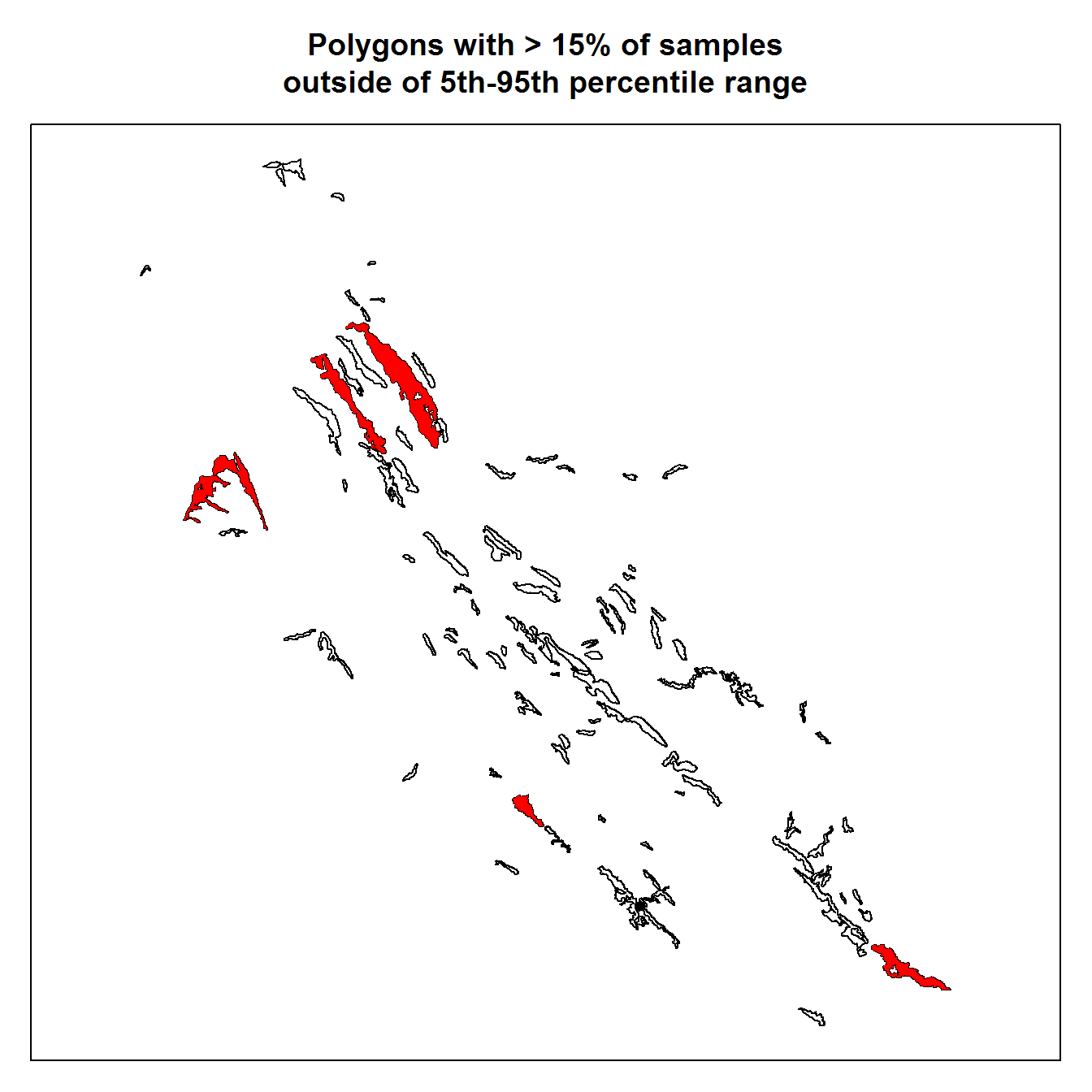Save flagged polygons to SHP file; be sure to adjust file paths. Note: `dsn` is the path to parent directory ("." means current working directory), and `layer` is the name of the shape file components--no need to specify ".shp".

``writeOGR(mu[check.idx, ], dsn='.', layer='flagged_polygons', driver='ESRI Shapefile', overwrite_layer=TRUE)``

Sort polygons based on similarity of sampled raster values.

``````# additional libraries
library(cluster)
library(MASS)

# eval numerical distance
p.dist <- daisy(poly.stats.wide[, -1], stand=TRUE)
# may need to tinker with number of clusters, 3 seems about right here
# cluster using divisive heirachical method
# the clustering vector is in the same order as our polygons
clusters <- cutree(diana(p.dist), 3)

# map distance matrix to 2D space via Sammon Mapping
p.sammon <- sammon(p.dist)

# plot
par(mar=c(1,1,3,1))
plot(p.sammon\$points, type='n', axes=FALSE, asp=1)
abline(h=0, v=0, lty=2, col='grey')
text(p.sammon\$points, labels = poly.stats.wide\$pID, cex=0.5, col=clusters)
box()
title('Polygon IDs Organized According to Similarity of Raster Values')``````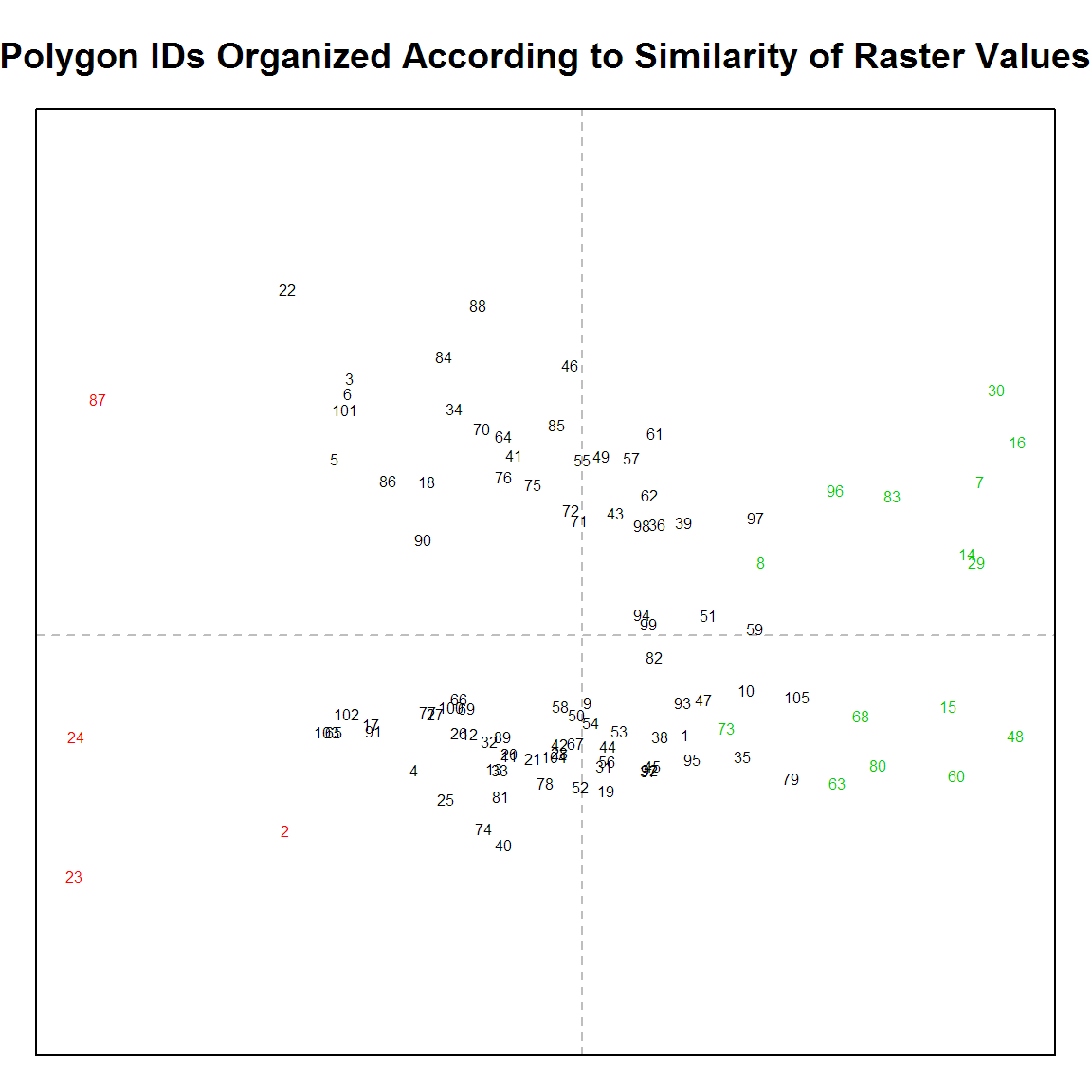``````# save clustering vector to original polygons, and map
mu\$cluster <- clusters
par(mar=c(1,1,3,1))
plot(mu, col=mu\$cluster, border=mu\$cluster)
box()
title('Polygons Grouped According to Similarity of Raster Values')``````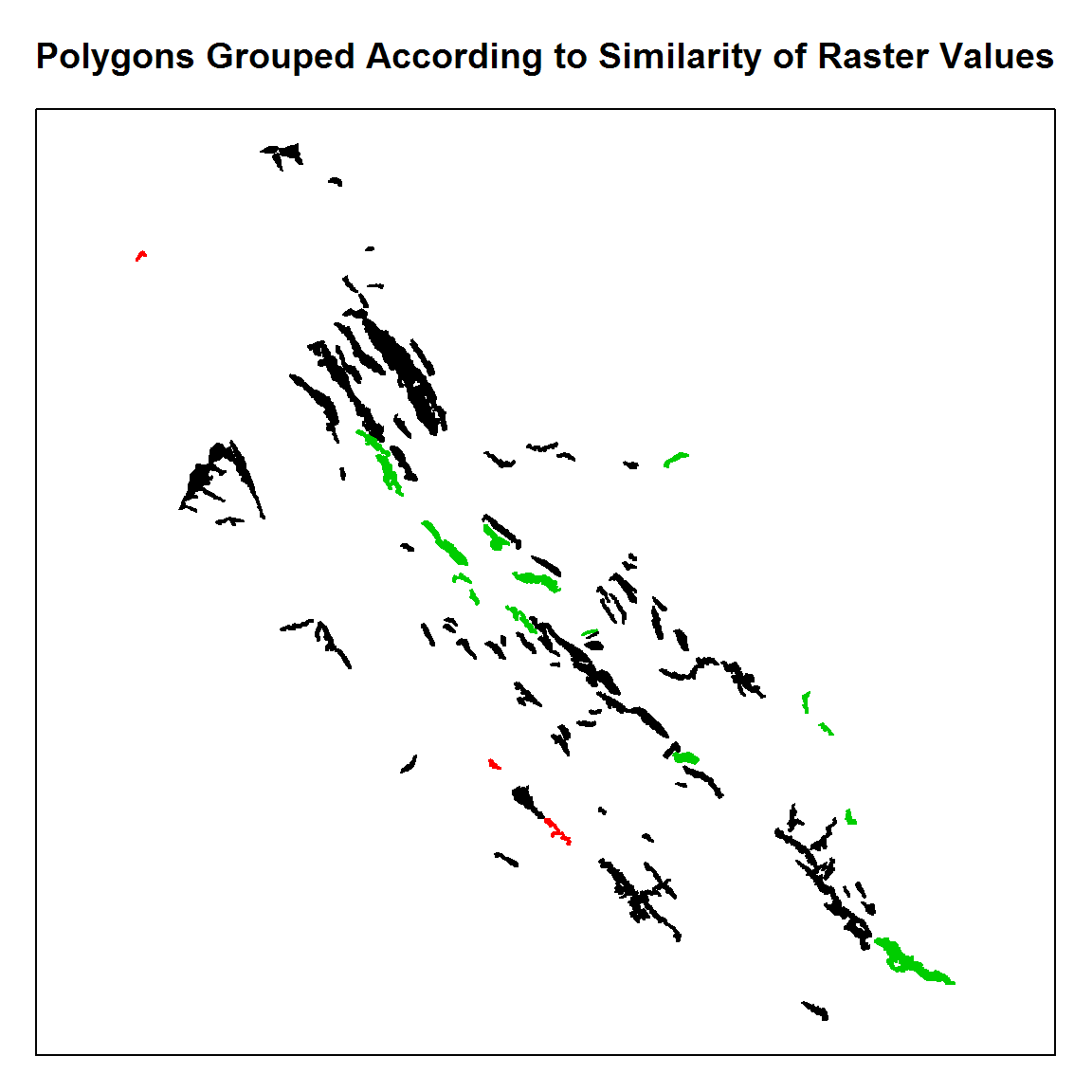This document is based on `sharpshootR` version 0.9.7.